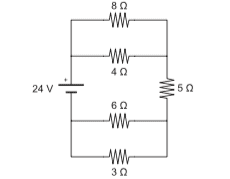# Potential difference of each resistor

GBC

## Homework StatementWhat is the potential difference across each resistor?

I know that I'll have to incorporate the V=iR equation
but I don't know where to start though...
My first attempt was with these:
R1=4 R2=8 R3=5 R4=6 R5=3
24-I1R1-I1R2-I3R3+I4R4+I5R5=0
but this lead me nowhere so I tried this
24-I1R1-I1R2-I2R3+I3R4+I4R5=0
and again, I was stuck so I tried making it into a simple series circuit by combining R1 with R2 and R4 with R5 and got this:
24-(8/3)I23-5I2+2I45=0
and again I was stuck...
How do I find the I-values for the respective resistor? Should I be finding each one or could I combine it, as I did in my last attempt? If I combine it, how would I be able to calculate the potential difference of each of the resistors in the combined pairs (R1/R2 and R4/R5)?

Homework Helper

## Homework Statement

View attachment 90370
What is the potential difference across each resistor?

I know that I'll have to incorporate the V=iR equation
but I don't know where to start though...
My first attempt was with these:
R1=4 R2=8 R3=5 R4=6 R5=3
24-I1R1-I1R2-I3R3+I4R4+I5R5=0
but this lead me nowhere so I tried this
24-I1R1-I1R2-I2R3+I3R4+I4R5=0
and again, I was stuck so I tried making it into a simple series circuit by combining R1 with R2 and R4 with R5 and got this:
24-(8/3)I23-5I2+2I45=0
and again I was stuck...
How do I find the I-values for the respective resistor? Should I be finding each one or could I combine it, as I did in my last attempt? If I combine it, how would I be able to calculate the potential difference of each of the resistors in the combined pairs (R1/R2 and R4/R5)?
Your first two trials have no sense. At the end you found out that the 4 ohm and 8 ohm resistors are connected parallel, and so are the 6 ohm and the 3 ohm resistors, and then you get three series connected resistors. So your last equation would be correct, What do you know of the current flowing through the series resistors? Can different current flow through them?